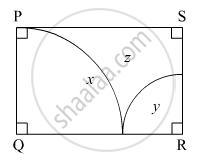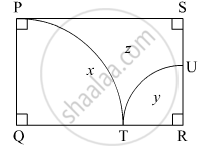SSC (Marathi Semi-English) 10thMaharashtra State Board
Share

# In the Given Figure, $\Square$ Pqrs is a Rectangle. If Pq = 14 Cm, Qr = 21 Cm, Find the Areas of the Parts X, Y And Z . - SSC (Marathi Semi-English) 10th - Geometry

#### Question

In the given figure,

$\square$ PQRS is a rectangle. If PQ = 14 cm, QR = 21 cm, find the areas of the parts x, y and z .#### Solution

PQRS is a rectangle.
∴ ∠Q = ∠R = 90ºRadius of sector PTQ = PQ = 14 cm
∴ Area of part x = Area of the sector PTQ =

$\frac{\theta}{360° } \times \pi r^2 = \frac{\angle Q}{360° } \times \pi \times \left( PQ \right)^2 = \frac{90° }{360° } \times \frac{22}{7} \times \left( 14 \right)^2$  = 154 cm2

Radius of sector TUR = TR = QR − QT = QR − PQ = 21 − 14 = 7 cm          (QT = PQ)
∴ Area of part y = Area of the sector TUR = $\frac{\theta}{360° } \times \pi r^2 = \frac{\angle R}{360° } \times \pi \times \left( TR \right)^2 = \frac{90°}{360° } \times \frac{22}{7} \times \left( 7 \right)^2$  = 38.5 cm

Now,
Area of rectangle PQRS = QR × PQ = 21 × 14 = 294 cm2
∴ Area of part z = Area of rectangle PQRS − Area of part x − Area of part y = 294 − 154 − 38.5 = 101.5 cm2

Is there an error in this question or solution?

#### APPEARS IN

Solution In the Given Figure, $\Square$ Pqrs is a Rectangle. If Pq = 14 Cm, Qr = 21 Cm, Find the Areas of the Parts X, Y And Z . Concept: Perimeter and Area of a Circle.
S